# The Basic Principle of 589nm ALL Solid State Laser

All-solid -state yellow lasers pumped by laser diode are developing quickly, and have great applications in laser medical, space target detection and identification, spectrum, laser display and other fields. At present, allsolid -state yellow lasers at 589nm with high power, high stability, high efficiency and high beam quality are theresearch interest in laser field. The research status and development of all-solid-state yellow lasers at 589nm were summarized and analyzed in this paper, which was useful reference for the future research in this field.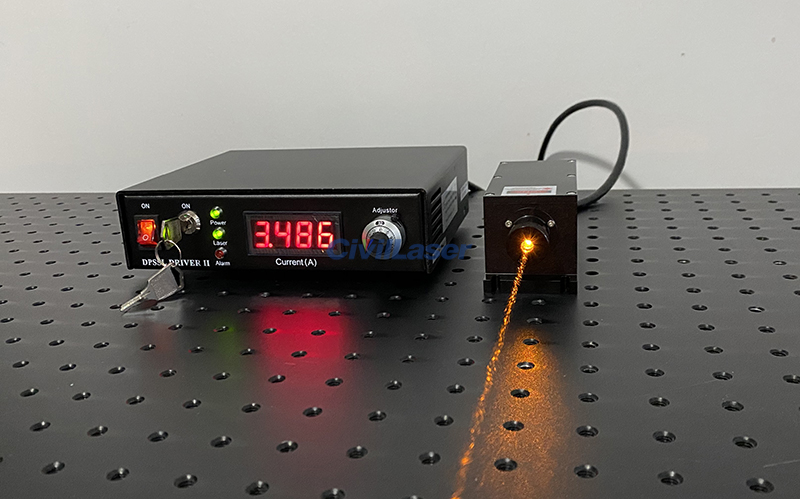Nd:YAG uses Nd3+ as the activated particle, and its main transition energy level diagram and emission cross-section parameters are shown in Figure 1, and Table 1.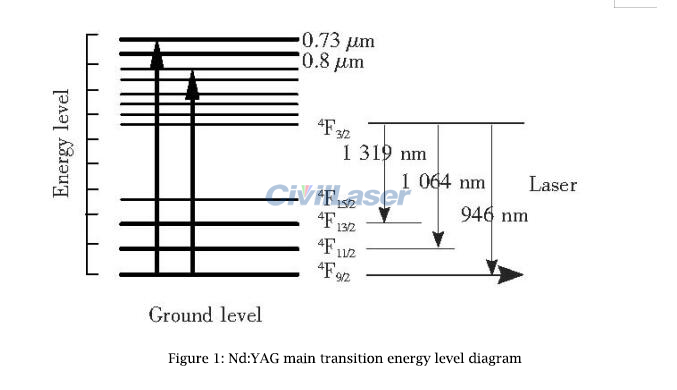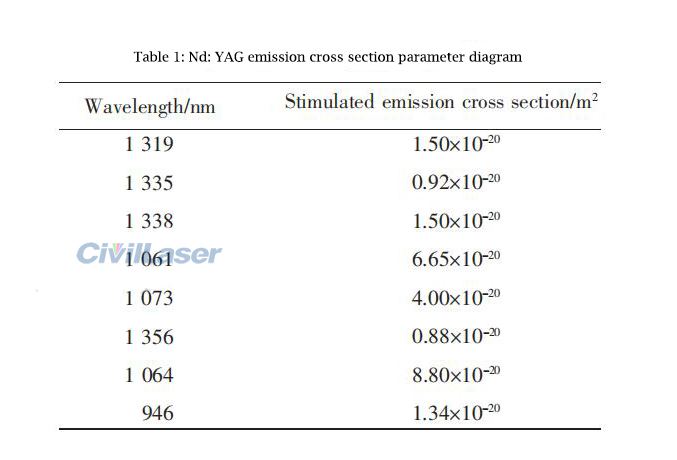The Nd3+ particles located at the energy level of the laser will undergo radiation transitions to the three terminal energy levels of 4F9/2, 4F11/2 and 4F13/2 at the emission branch ratio of 0.6:0.25:0.14, emitting 946nm, 1064nm and respectively. 1 319nm fluorescence spectrum. Among them, 1064nm and 1 319nm belong to a four-level system with a low threshold, and it is easy to obtain laser output at room temperature. The 589nm yellow laser is obtained by summing the 1064nm and 1319nm lasers. In order to effectively transfer energy from pumping waves with wavelengths of 1064nm and 1319nm to generated waves with wavelengths of 589nm, the conservation of energy and momentum must be satisfied during the sum frequency process, where energy conservation can be expressed as: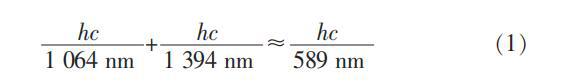Among them, ‘h’ is Planck’s constant and ‘c’ is the speed of light.

In order to obtain higher sum frequency efficiency, the power of the two fundamental frequency lights needs to meet a certain ratio. In an ideal state, a 1064nm photon and a 1 319nm photon undergo a nonlinear transformation to produce a 589nm photon. Therefore, the theoretical optimal ratio of the power of 1064nm laser and 1319nm laser in the cavity should be: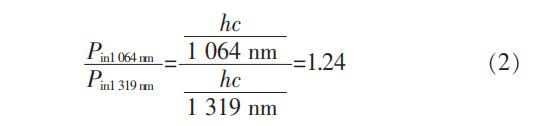It is also known that the power in the cavity can be calculated by the following formula: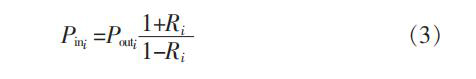Among them, Pout and R represent the laser output power and the reflectance of the coupling-out mirror respectively, and the angle scale i=1064nm or 1319nm, which corresponds to the parameters of the 1 064nm and 1319nm lasers, respectively. By selecting the appropriate LD pumping power ratio of the two lasers, it is possible to ensure that the two fundamental frequency beams in the cavity achieve the best power ratio on the nonlinear crystal, thereby improving the sum frequency efficiency.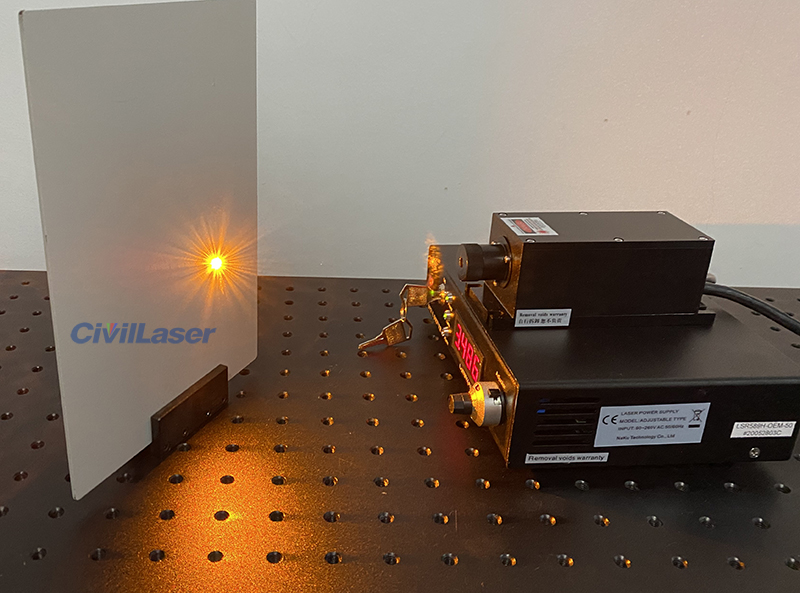Close
Close